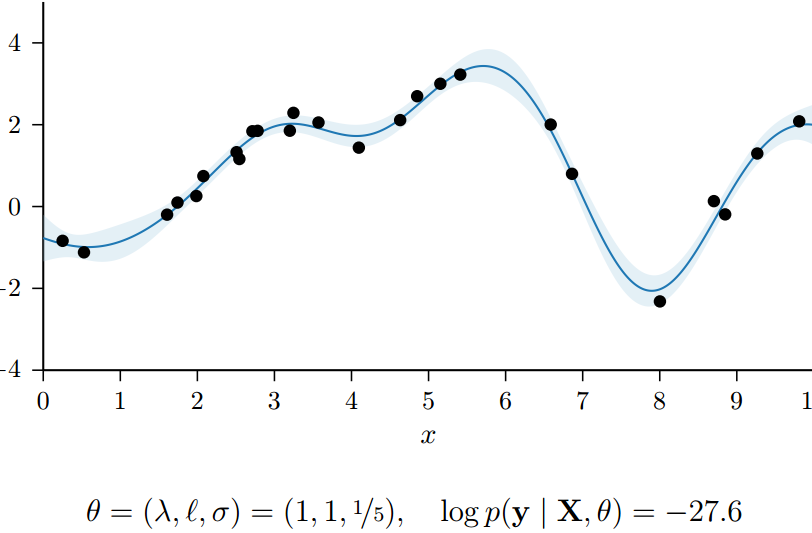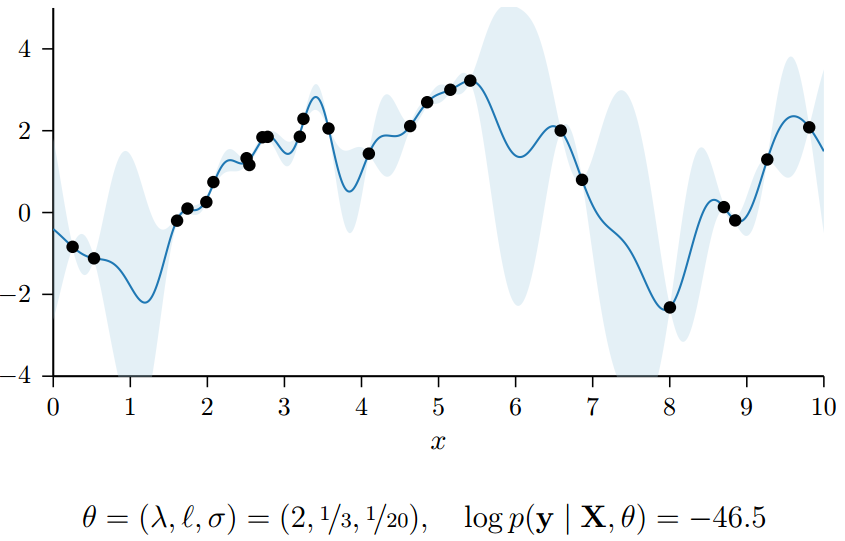# Gaussian Process Regression

## From Bayesian Linear Regression to Gaussian Process

Posted by HC on March 11, 2018

# 1. Linear Regression

​ 线性回归问题是机器学习中最为基础的一个回归模型。线性回归使用了square loss，旨在使用一个线性函数$f(X)=X\beta$来拟合当前数据。为了简单方便，这里不考虑kernel extension的情况。其优化目标$L(Y,f(X))$和solution可以写作下式。这里假设solution $\beta$中的$X^TX$是可逆的（否则可以考虑GD的方式求解，或者做特征选择，做降维，或者考虑加入L2范数，以去掉trivial solution）。这里的$\widehat{Y}=X\beta$是响应变量$Y$在数据$X$的feature列空间下的正交投影。

$L(Y, f(X))=(Y-X\beta)^T(Y-X\beta) \\ \beta=(X^TX)^{-1}X^TY$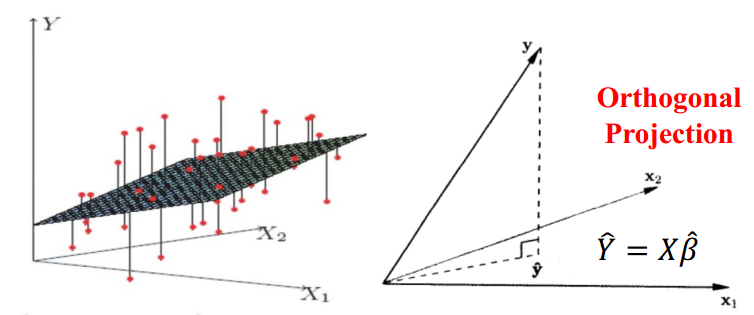# 2. Bayesian Linear Regression

$W \sim N(\mu, \Sigma)\\ f \sim N(X\mu, X\sigma X^T) \\ y \sim N(X\mu, X\Sigma X^T+\sigma^2I)$

$P(W,y \mid X,\mu.\sigma^2,\Sigma)=N \left(\begin{bmatrix} \mu \\ X\mu \end{bmatrix},\begin{bmatrix} \Sigma & (X\Sigma)^T\\ X\Sigma & X\Sigma X^T + \sigma^2I \end{bmatrix} \right) \\ cov(y,W)=cov(XW+\varepsilon, W) = cov(XW,W)=X\Sigma$

$P(W\mid X,y, \mu, \Sigma,\sigma^2)=N(\mu_W,\Sigma_W)\\ \mu_W=\mu + \Sigma X^T (X \Sigma X^T+\sigma^2I)^{-1}(y-X\mu)\\ \Sigma_W=\Sigma-\Sigma X^T(X\Sigma X^T + \sigma^2I)^{-1} X \Sigma$

$P(y^0 |X,y,X^0 ,\mu,\Sigma,\sigma^2)\\ =\int P(y^0 \mid W, X^0 , \sigma^2)P(W \mid X,y,\mu,\Sigma,\sigma^2)dW\\ =N(X^0 \mu_W,X^0 \Sigma_W (X^0 )^T+\sigma^2I)$

# 3. Gaussian Process Regression

​ GPR可以算作是比贝叶斯线性回归更加general的一个模型。GPR是说，与其想参数$W$的先验，不如就直接想回归函数的先验分布。所以GPR的先验不是定义在参数上，而是模型本身。那么为啥不直接考虑参数$W$的先验呢？原因是我们模型$f$是潜在的无穷维度的，而以前的方式在有限维度上work。所以大牛们说：The Gaussian process is a natural generalization of the multivariate Gaussian distribution to potentially infinite settings

​ 对于GP的正式定义，这里就不写（抄）了。简单来说，GP可以由mean function $\mu(X)$和covariance function（或者叫kernel） $K(X,X)$唯一确定。可以写成：

$$P(f\mid X)=N(\mu(X),K(X,X))$$ 其中，

• mean function度量了函数的central tendency，一般来说是一个常数，比如0。当然也可以用其他函数，考虑到会引入额外参数，一般0是比较常用的选择
• kernel表达了我们希望function具有的性质和形状，比如常用的高斯径向基核函数就想要靠的近的点有相似的函数值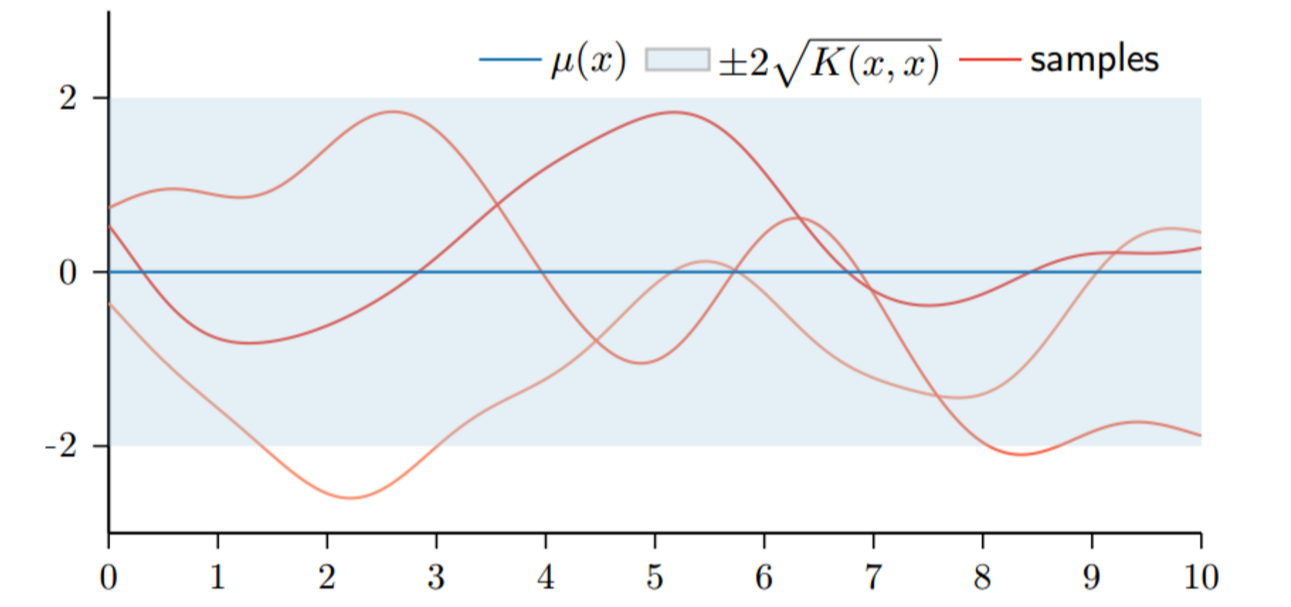​ 那么GPR是怎么预测数据的呢？这个过程和之前的Bayesian Linear Regression类似，是从联合概率推后验概率。给定已有的训练数据$X$，回归值$f$ 和test data $X^0$，我们要求预测值$f^0 =f(X^0 )$。那么联合概率可以表示为：

$P(f,f^0 )=N \left(\begin{bmatrix} \mu(X) \\ X\mu(X^0 ) \end{bmatrix},\begin{bmatrix} K(X,X) & K(X,X^0 )\\ K(X^0 ,X) & K(X^0 ,X^0 ) \end{bmatrix} \right)$

​ 那么，进一步得到条件概率的形式。需要一说的是，GPR也是可以考虑noise的情况的，这里没有再做推导，具体方法可以参考Bayesian Linear Regression。（下面的公式中的$K^{-1}=K(X, X)^{-1}$，或者考虑有高斯noise，$K^{-1}=(K(X, X)+\sigma^2I)^{-1}$）。这个解的形式，和之前的Bayesian Ridge Regression的形式是很相似的，实际上，当mean function等于0，kernel取值$K=XX^T$的时候，两个模型是等价的

$P(f^0 \mid X^0 , X,f)=N(\mu_{0}(X^0 ),K_{0}(X^0 ,X^0 ))\\ \mu_{0}(X^0 )=\mu(X^0 )+K(X^0 ,X)K^{-1}(f-\mu(X))\\ K_{0}(X^0 ,X^0 )=K(X,X^0 )-K(X^0 ,X)K^{-1}K(X,X^0 )$

​ 事实上，对于GP来说，他的后验均值可以看做是weighted combination of kernel function ，这里的weight $\alpha_{i}=K(X,X)^{-1}(f(X_{i}-\mu(X_{i})))$

$\mu_{0}(X^0 )=\mu(X^0 )+K(X^0 ,X)K^{-1}(f-\mu(X))\\ =\mu(X^0 ) + \sum^N_{i=1} \alpha_iK(X_i,X^0 )$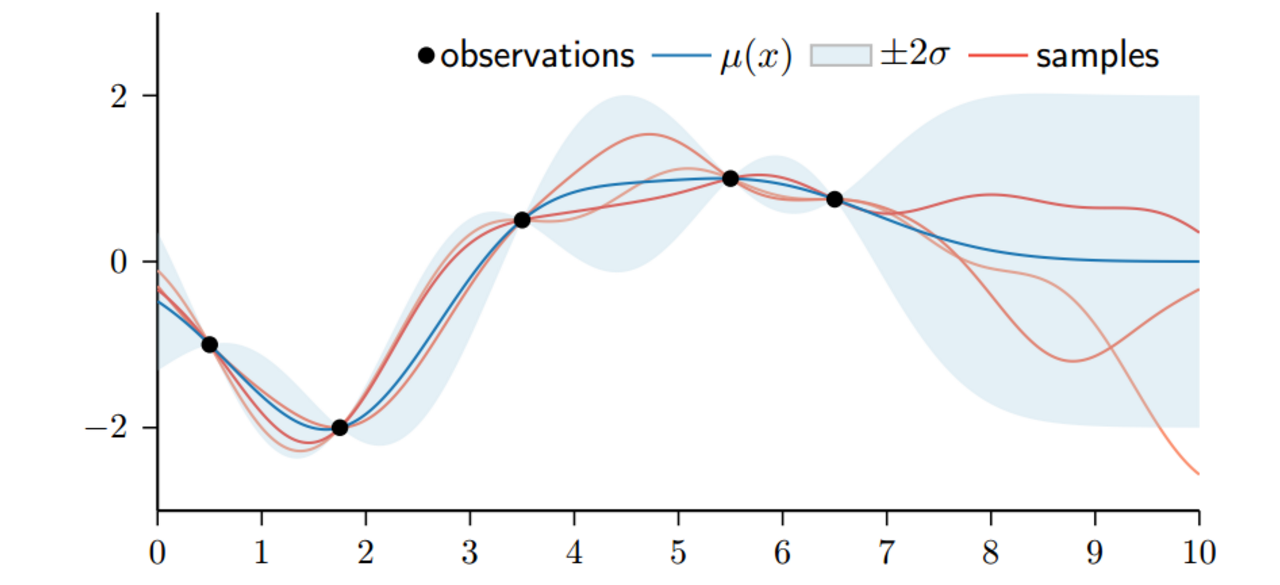​ 注意到图里有阴影部分，这个是表示预测的方差（置信度）。对于存在数据的地方，预测方差很小，否则不确定性越大。这里也是GPR有一个优于贝叶斯线性回归的地方，GPR的后验分布直接能给出预测方差，而不需要借助predictive distribution遍历参数空间做一个加权和。当然GPR的求解会涉及到（增量式）矩阵求逆，这个操作是很耗时的，是GPR的一个大bug。

# 4. Hyper-parameters of GPR

GP的kernel选择有很多，可以是周期性的，也可以是非周期性的。但是核都是有参数的。所以对于GPR来说，参数来自于kernel和mean function。一般考虑mean function=0，那么模型参数来自于kernel function，比如length scale, the output scale, and the noise variance，现在把参数统记为$\theta$。为了要学习超参，需要使用marginal likelihood，他是用来度量在给定先验的情况下，拟合数据的好坏层度。

$P(y\mid X,\theta)=\int P(y\mid f)P(f\mid X, \theta)df$

$P(y\mid X,\theta)=N(\mu(X;\theta),K(X,X;\theta)+\sigma^2I)$

$log P(y \mid X, \theta)=-\frac{(y-\mu)^TV^{-1}(y-\mu)}{2}-\frac{log\mbox{ } detV}{2} -\frac{Nlog2\pi}{2}$

1. 第一项代表了data fitness。是在V度量下的距离度量（均值和真实值y），所有距离越小越好，然后还有个负号，所以越大越好
2. 第二项是Occam’s razor，有负号，所以也是越大越好。等价于det V越小越好，由于噪声的方差实际中没法控制，所以，考虑gp的方差越小越好，模型变化范围小，能表达数据的能力小，模型趋于简单。
3. 最后当然是数据越多越好了